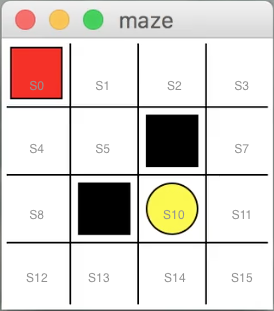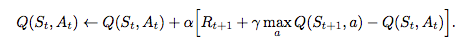AI学习笔记——Q Learning

0
0
01. 云栖社区>
2. 博客>
3. 正文

AI学习笔记——Q Learning

hongtao2018 2018-08-12 06:55:00 浏览470

• 状态(State (S))
• 动作(Action(A))
• 奖励(Reward(R))1. Q表(Q table)

Q Learning 就是创造一个Q表，来指导机器人的行动，Q表对应Action的数值越大，机器人就越大概率地采取这个Action.

Q table (States\Actions) left (A1) right (A2) up (A3) down (A4)
S0 -1 3 -1 2
S1 1 2 -1 1
... ... ... ... ..

2. 探索-利用困境(Explore-Exploit dilemma)

Q Learning 是如何学习并更新Q表呢？正如多臂老虎机(Multi-armed bandit)问题一样，如果机器人仅仅按照表中最大概率指导行动的话，是学不到任何东西的，它还需要不停地在未知地图上进行探索，这就是是探索-利用困境(Explore-Exploit dilemma)。同样的，我们也可以用ε贪婪方法(ε -Greedy method)的方法来解决这个困境。

3. 如何更新Q表

Q learning的算法就是如何更新Q表的方法。还是以表下为例：

Q table (States\Actions) left (A1) right (A2) up (A3) down (A4)
S0 -1 3 -1 2
S1 1 2 -1 1

Q(S0,A2) = Q(S0,A2) + α[R(S1) + γ*maxa Q(S1,a)-Q(S0,A2)]

• R(S1)是机器人在S1能活的的奖励(Reward)(该游戏除了陷阱的地方为-1，宝藏的地方为1，其他地方均为0)。
• γ为衰减值。
• maxa Q(S1,a)是S1状态下Q表数值最大的一个(这里是2)。
• α是学习速率(Learning Rate)。
• R(S1) + γ*maxa Q(S1,a)是Q(S0,A2) 的目标数值。

Q(S0,A2)新=Q(S0,A2) 旧 + α* [Q(S0,A2)目标 - Q(S0,A2)旧]hongtao2018
+ 关注

corcosa 11208人浏览